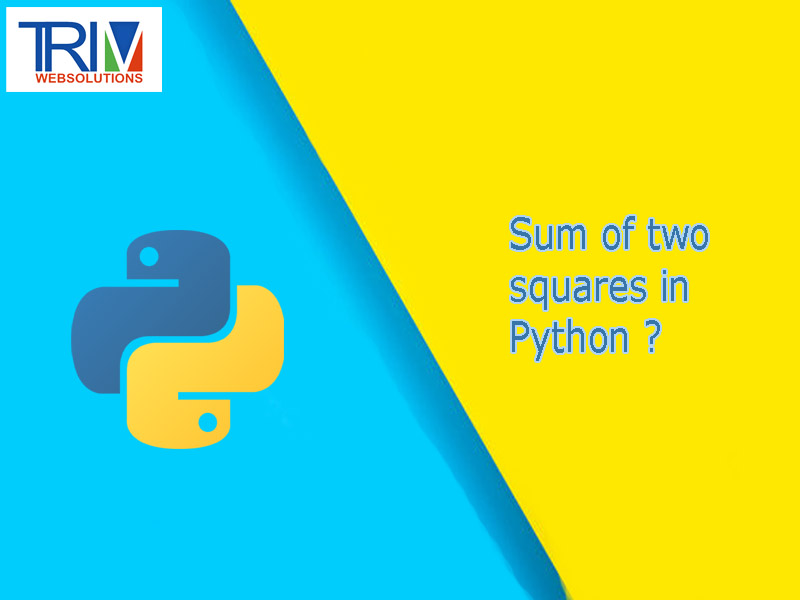Quick Inquiry

# Sum of two squares in Python ?You do not need the ranges at all, and certainly do not need to convert them into tuples. They take a ridiculous amount of space, but you only need their current elements, numbers `i` and `j`. Also, as the friendly commenter suggested, you can start with `sqrt(n)` to improve the performance further.

``` ```def sum_of_two_squares(n):
i = 1
j = int(n ** 1/2)

while i < j:
x = i * i + j * j
if x == n:
return j, i

if x < n:
i += 1
else:
j -= 1``````